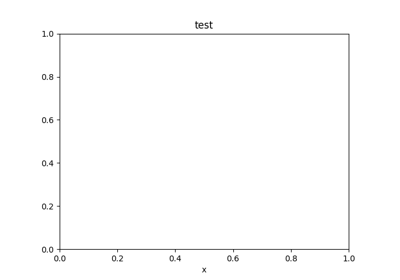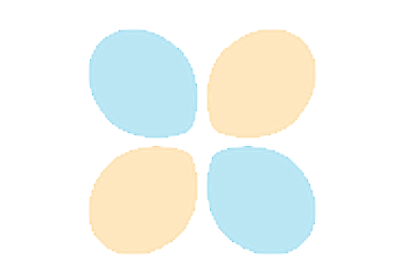Travis-CI:# matplotlib.pyplot.pcolor¶

`matplotlib.pyplot.``pcolor`(*args, **kwargs)

Create a pseudocolor plot of a 2-D array.

Call signatures:

```pcolor(C, **kwargs)
pcolor(X, Y, C, **kwargs)
```

pcolor can be very slow for large arrays; consider using the similar but much faster `pcolormesh()` instead.

Parameters:

C : array_like

An array of color values.

X, Y : array_like, optional

If given, specify the (x, y) coordinates of the colored quadrilaterals; the quadrilateral for `C[i,j]` has corners at:

```(X[i,   j],   Y[i,   j]),
(X[i,   j+1], Y[i,   j+1]),
(X[i+1, j],   Y[i+1, j]),
(X[i+1, j+1], Y[i+1, j+1])
```

Ideally the dimensions of `X` and `Y` should be one greater than those of `C`; if the dimensions are the same, then the last row and column of `C` will be ignored.

Note that the column index corresponds to the x-coordinate, and the row index corresponds to y; for details, see the Grid Orientation section below.

If either or both of `X` and `Y` are 1-D arrays or column vectors, they will be expanded as needed into the appropriate 2-D arrays, making a rectangular grid.

cmap : `Colormap`, optional, default: None

If `None`, default to rc settings.

norm : `matplotlib.colors.Normalize`, optional, default: None

An instance is used to scale luminance data to (0, 1). If `None`, defaults to `normalize()`.

vmin, vmax : scalar, optional, default: None

`vmin` and `vmax` are used in conjunction with `norm` to normalize luminance data. If either is `None`, it is autoscaled to the respective min or max of the color array `C`. If not `None`, `vmin` or `vmax` passed in here override any pre-existing values supplied in the `norm` instance.

edgecolors : {None, ‘none’, color, color sequence}

If None, the rc setting is used by default. If ‘none’, edges will not be visible. An mpl color or sequence of colors will set the edge color.

alpha : scalar, optional, default: None

The alpha blending value, between 0 (transparent) and 1 (opaque).

snap : bool, optional, default: False

Whether to snap the mesh to pixel boundaries.

Returns:

collection : `matplotlib.collections.Collection`

Other Parameters:

antialiaseds : bool, optional, default: False

The default `antialiaseds` is False if the default `edgecolors="none"` is used. This eliminates artificial lines at patch boundaries, and works regardless of the value of alpha. If `edgecolors` is not “none”, then the default `antialiaseds` is taken from `rcParams['patch.antialiased']`, which defaults to True. Stroking the edges may be preferred if `alpha` is 1, but will cause artifacts otherwise.

**kwargs :

Any unused keyword arguments are passed along to the `PolyCollection` constructor:

Property Description
`agg_filter` a filter function, which takes a (m, n, 3) float array and a dpi value, and returns a (m, n, 3) array
`alpha` float or None
`animated` bool
`antialiased` or antialiaseds Boolean or sequence of booleans
`array` ndarray
`clim` a length 2 sequence of floats
`clip_box` a `Bbox` instance
`clip_on` bool
`clip_path` [(`Path`, `Transform`) | `Patch` | None]
`cmap` a colormap or registered colormap name
`color` matplotlib color arg or sequence of rgba tuples
`contains` a callable function
`edgecolor` or edgecolors matplotlib color spec or sequence of specs
`facecolor` or facecolors matplotlib color spec or sequence of specs
`figure` a `Figure` instance
`gid` an id string
`hatch` [ ‘/’ | ‘' | ‘|’ | ‘-‘ | ‘+’ | ‘x’ | ‘o’ | ‘O’ | ‘.’ | ‘*’ ]
`label` object
`linestyle` or dashes or linestyles [‘solid’ | ‘dashed’, ‘dashdot’, ‘dotted’ | (offset, on-off-dash-seq) | `'-'` | `'--'` | `'-.'` | `':'` | `'None'` | `' '` | `''`]
`linewidth` or linewidths or lw float or sequence of floats
`norm` `Normalize`
`offset_position` [ ‘screen’ | ‘data’ ]
`offsets` float or sequence of floats
`path_effects` `AbstractPathEffect`
`picker` [None | bool | float | callable]
`pickradius` float distance in points
`rasterized` bool or None
`sketch_params` (scale: float, length: float, randomness: float)
`snap` bool or None
`transform` `Transform`
`url` a url string
`urls` List[str] or None
`visible` bool
`zorder` float

`pcolormesh`
for an explanation of the differences between pcolor and pcolormesh.

Notes

`X`, `Y` and `C` may be masked arrays. If either C[i, j], or one of the vertices surrounding C[i,j] (`X` or `Y` at [i, j], [i+1, j], [i, j+1], [i+1, j+1]) is masked, nothing is plotted.

The grid orientation follows the MATLAB convention: an array `C` with shape (nrows, ncolumns) is plotted with the column number as `X` and the row number as `Y`, increasing up; hence it is plotted the way the array would be printed, except that the `Y` axis is reversed. That is, `C` is taken as `C` (y, x).

Similarly for `meshgrid()`:

```x = np.arange(5)
y = np.arange(3)
X, Y = np.meshgrid(x, y)
```

is equivalent to:

```X = array([[0, 1, 2, 3, 4],
[0, 1, 2, 3, 4],
[0, 1, 2, 3, 4]])

Y = array([[0, 0, 0, 0, 0],
[1, 1, 1, 1, 1],
[2, 2, 2, 2, 2]])
```

so if you have:

```C = rand(len(x), len(y))
```

then you need to transpose C:

```pcolor(X, Y, C.T)
```

or:

```pcolor(C.T)
```

MATLAB `pcolor()` always discards the last row and column of `C`, but Matplotlib displays the last row and column if `X` and `Y` are not specified, or if `X` and `Y` have one more row and column than `C`.

Note

In addition to the above described arguments, this function can take a data keyword argument. If such a data argument is given, the following arguments are replaced by data[<arg>]:

• All positional and all keyword arguments.

## Examples using `matplotlib.pyplot.pcolor`¶Simple animation examplesSaving an animation